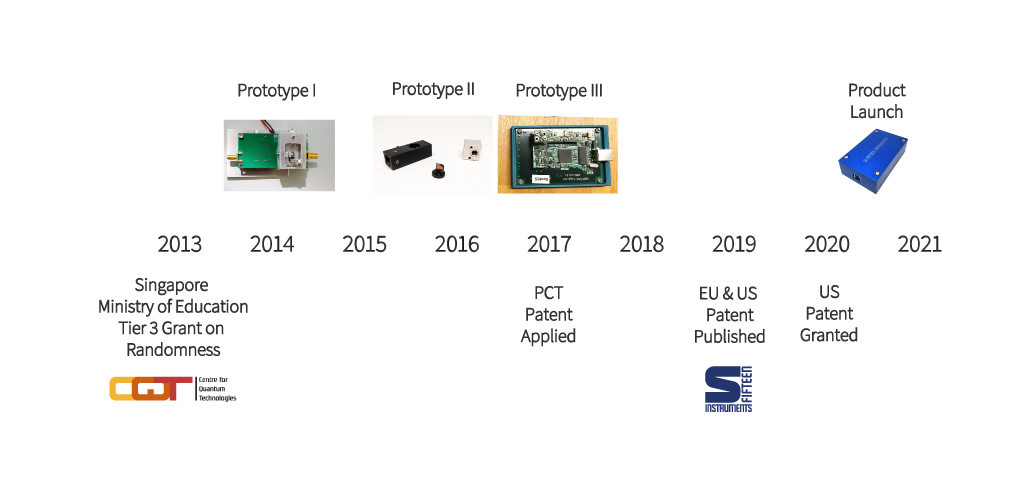0

# Why are random numbers important?

To secure digital communication, we require cryptographic schemes. In both classical and quantum versions, the generation of keys and encryption protocols rely on random numbers. In order to keep up with data rates in modern communication schemes, these random numbers also need to be generated at a high rate. The choice of source of random numbers even determines how a cryptographic scheme can be attacked.

Another important application of random numbers in computing is for numerical simulation. For example, in Monte Carlo calculations the quality of random numbers determines the accuracy of the simulation result and the speed of generation determines whether a complicated simulation can be completed in a reasonable amount of time.

# Where do we get good random numbers from?

The easiest way to generate random numbers is to use a computational algorithm with a seemingly random output. However, these pseudo-random numbers are deterministic by definition and are unsuitable for cryptographic purposes, as they may contain backdoors in the particular algorithm used to generate them.

Physical random number generators measure noisy physical processes and convert the outcome into random numbers. Since it is either practically (e.g. for thermal noise sources) or fundamentally (for certain quantum processes) impossible to predict the outcome of such measurements, these physically generated random numbers are considered “truly” random.

### Comparison of Sources of Random Numbers

 Type of Random Number Generator Entropy Source Example "Truly" Random? Pseudo Trusted random bit sequence as the seed glibc's random() function No Physical Measurement of a physical process whose outcome is determined by classical mechanics /dev/random in linux kernel Yes* Physical Measurement of a fundamentally probabilistic quantum process S-Fifteen's QRNG1 Yes**

*In so far you are unable to access all parameters that determine the outcome of the measurement (in practice not possible).
**In so far that you trust Quantum Theory which to date has not been refuted experimentally.

# Not a Black Box

Our approach is to perform measurements of a quantum noise source and utilize an efficient randomness extractor to remove the residual bias or correlations and thus generate "truly" random numbers at a high rate. The source of quantum noise are the vacuum fluctuations of the electromagnetic field in a certain mode which are intrinsically unpredictable, and inaccessible to an adversary.

In our device, we measure the vacuum fluctuations of the electromagnetic field via a homodyne technique which involves superimposing the vacuum field with a local oscillator (laser beam). The measurement output is passed on to a randomness extractor which produces an output stream of numbers with a uniform distribution at a reduced rate.Unlike typical randomness extractors based on cryptographic hashing functions which are slow due to their complexity, our Linear Feedback Shift Register (LFSR) based extractor is significantly fast and lean on resources. The LFSR-based extractor does not sacrifice any of the security of cryptographic hashing and is shown to be equivalent to the Toeplitz matrix hashing function.

Our device also integrates a self-testing function to continuously monitor the quality of the output random numbers which satisfy standard statistical test suites (NIST SP 800-22 and Dieharder). We have integrated the optics and electronics into a compact device that fits in the palm of your hand and outputs a random bit stream of about 480 Mbits/s, currently limited by the USB2 serial bus speed.